# Chapter 8 Functions Functions l Every C program

• Slides: 49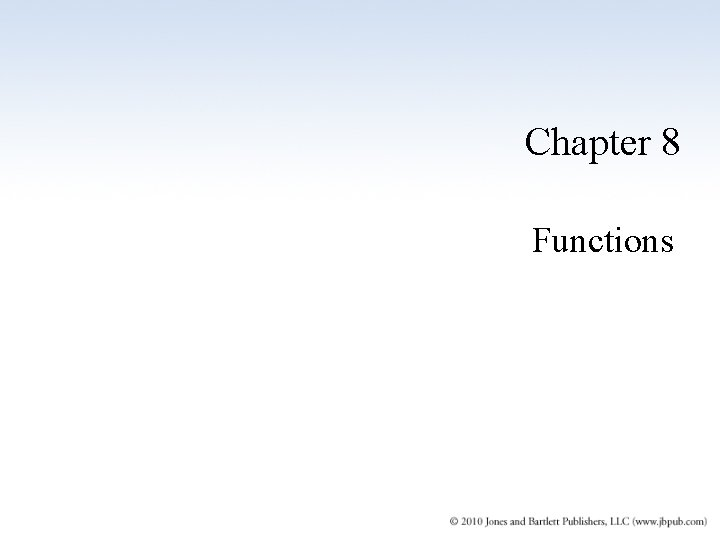Chapter 8 FunctionsFunctions l Every C++ program must have a function called main l Program execution always begins with function main l Any other functions are subprograms that must be explicitly called 2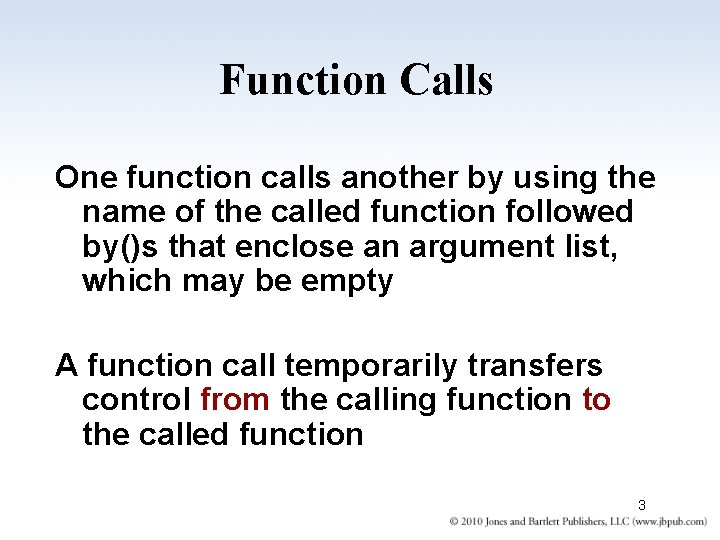Function Calls One function calls another by using the name of the called function followed by()s that enclose an argument list, which may be empty A function call temporarily transfers control from the calling function to the called function 3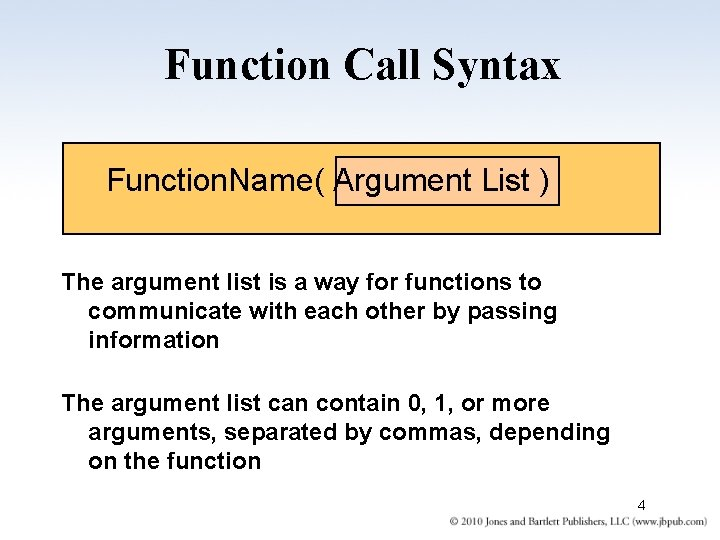Function Call Syntax Function. Name( Argument List ) The argument list is a way for functions to communicate with each other by passing information The argument list can contain 0, 1, or more arguments, separated by commas, depending on the function 4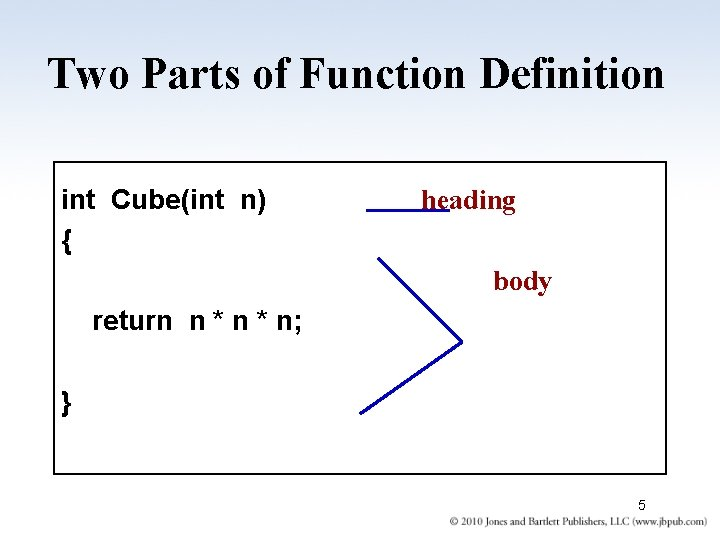Two Parts of Function Definition int Cube(int n) { heading body return n * n; } 5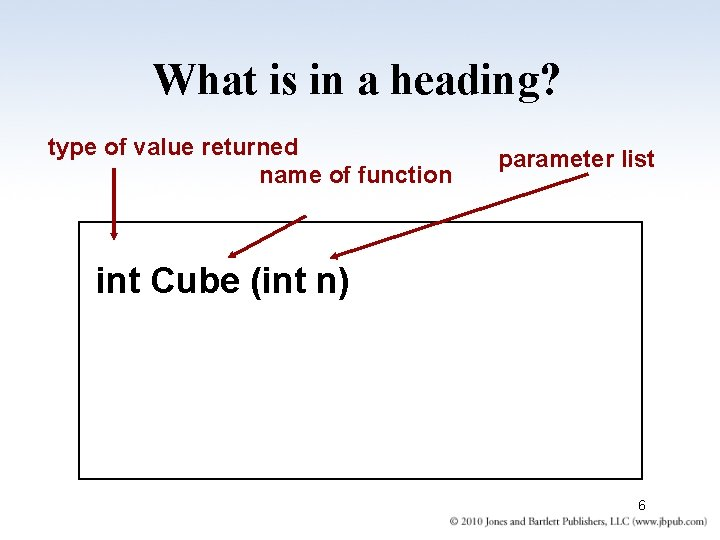What is in a heading? type of value returned name of function parameter list int Cube (int n) 6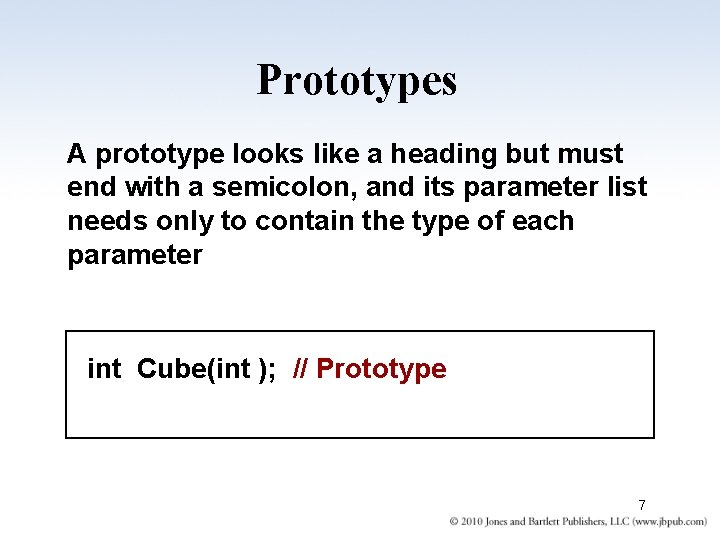Prototypes A prototype looks like a heading but must end with a semicolon, and its parameter list needs only to contain the type of each parameter int Cube(int ); // Prototype 7Function Calls When a function is called, temporary memory is allocated for its value parameters, any local variables, and for the function’s name if the return type is not void Flow of control then passes to the first statement in the function’s body The called function’s statements are executed until a return statement (with or without a return value) or the closing brace of the function body is encountered Then control goes back to where the function was called 8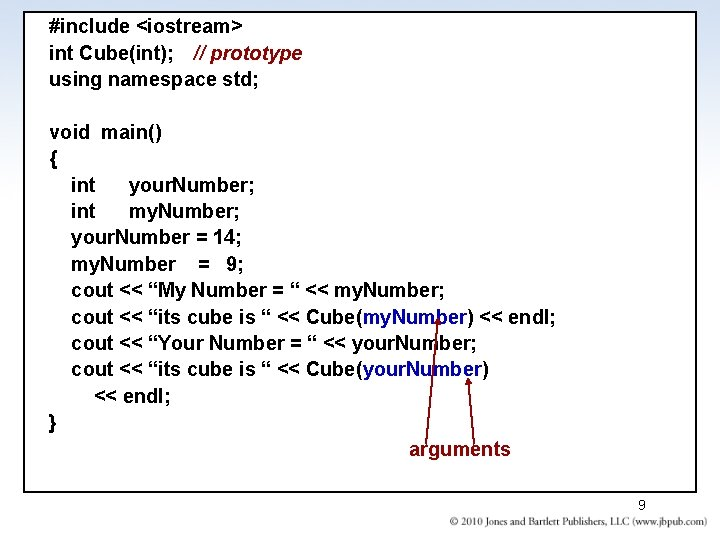#include <iostream> int Cube(int); // prototype using namespace std; void main() { int your. Number; int my. Number; your. Number = 14; my. Number = 9; cout << “My Number = “ << my. Number; cout << “its cube is “ << Cube(my. Number) << endl; cout << “Your Number = “ << your. Number; cout << “its cube is “ << Cube(your. Number) << endl; } arguments 9Successful Function Compilation Before a function is called in your program, the compiler must have previously processed either the function’s prototype or the function’s definition (heading and body) 10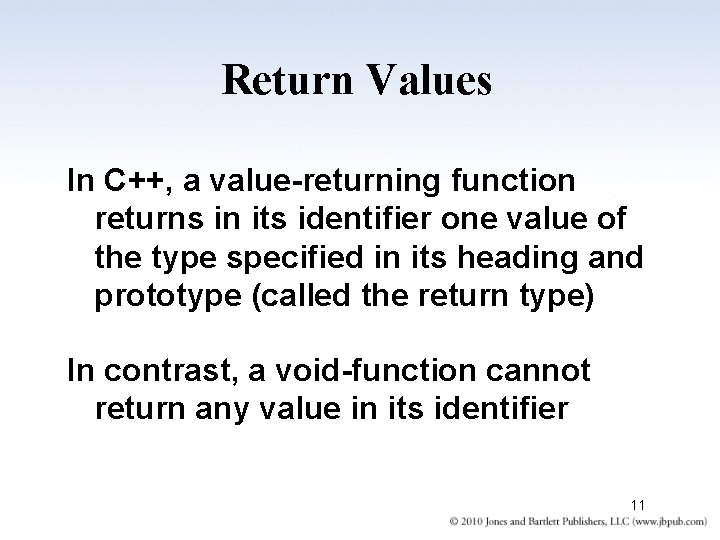Return Values In C++, a value-returning function returns in its identifier one value of the type specified in its heading and prototype (called the return type) In contrast, a void-function cannot return any value in its identifier 11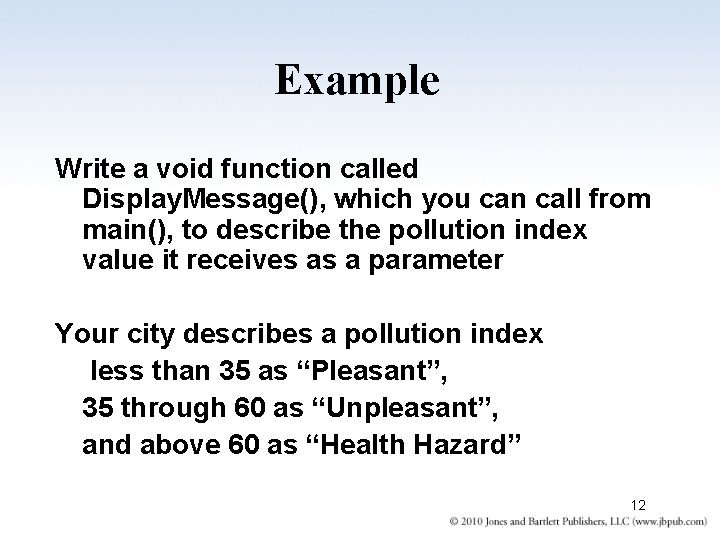Example Write a void function called Display. Message(), which you can call from main(), to describe the pollution index value it receives as a parameter Your city describes a pollution index less than 35 as “Pleasant”, 35 through 60 as “Unpleasant”, and above 60 as “Health Hazard” 12parameter void Display. Message(int index) { if(index < 35) cout << “Pleasant”; else if(index <= 60) cout << “Unpleasant”; else cout << “Health Hazard”; } 13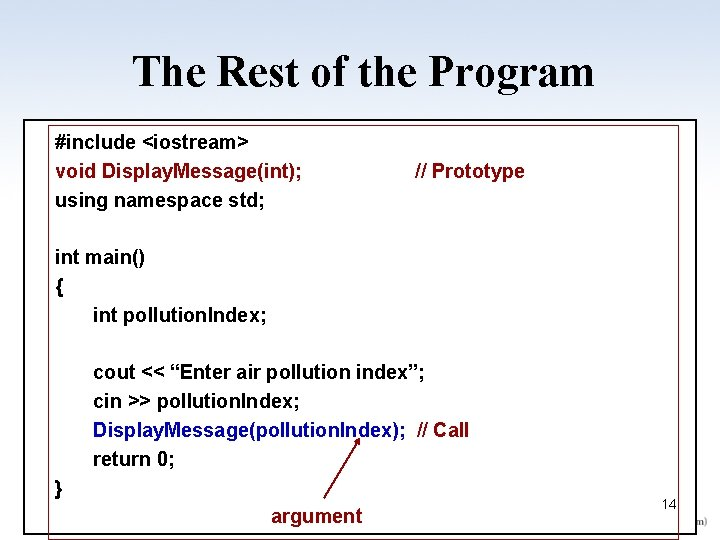The Rest of the Program #include <iostream> void Display. Message(int); using namespace std; // Prototype int main() { int pollution. Index; cout << “Enter air pollution index”; cin >> pollution. Index; Display. Message(pollution. Index); // Call return 0; } argument 14 14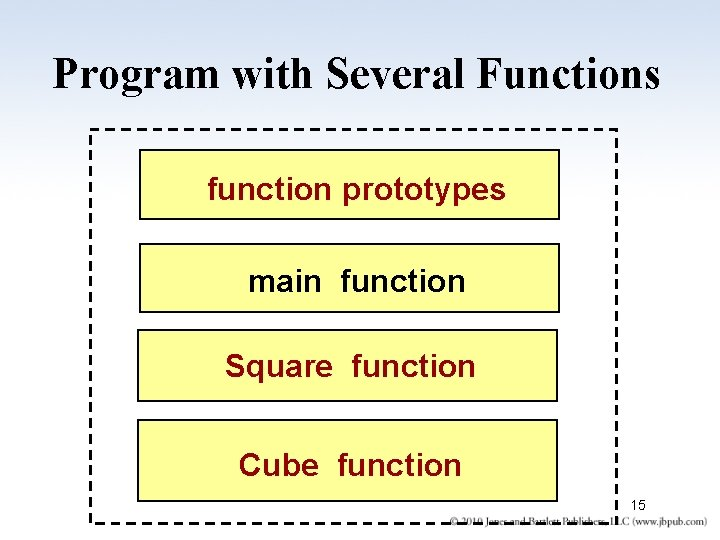Program with Several Functions function prototypes main function Square function Cube function 15Value-Returning Functions #include <iostream> int Square(int); int Cube(int); using namespace std; // Prototypes int main() { cout << “The square of 27 is “ << Square(27) << endl; cout << “The cube of 27 is “ << Cube(27) << endl; return 0; } function calls 16 16Rest of Program int Square(int n) { return n * n; } // Header and body int Cube(int n) { return n * n; } // Header and body 17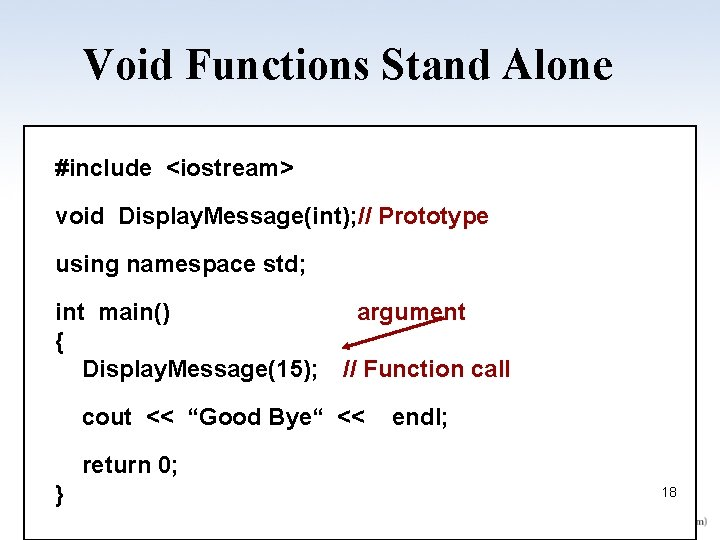Void Functions Stand Alone #include <iostream> void Display. Message(int); // Prototype using namespace std; int main() { Display. Message(15); argument // Function call cout << “Good Bye“ << endl; return 0; } 18 18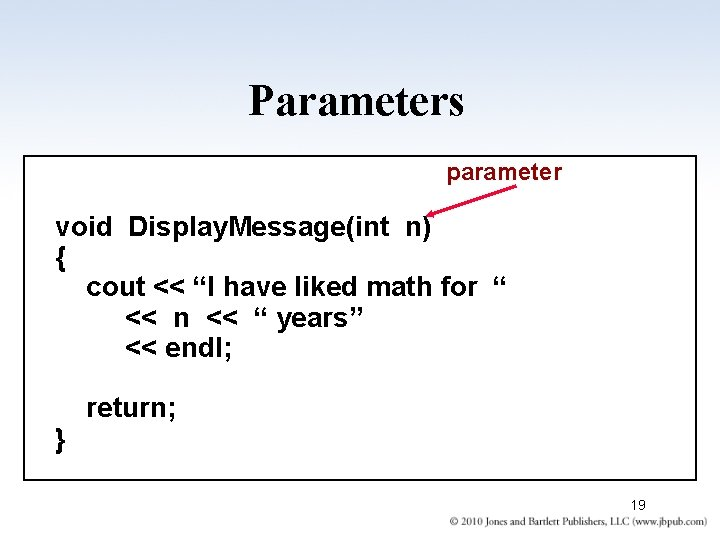Parameters parameter void Display. Message(int n) { cout << “I have liked math for “ << n << “ years” << endl; } return; 19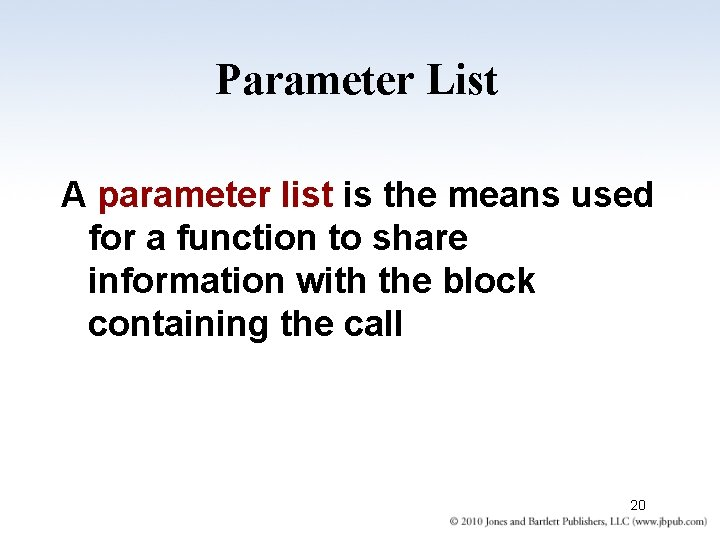Parameter List A parameter list is the means used for a function to share information with the block containing the call 20Classified by Location Arguments Always appear in a function call within the calling block Parameters Always appear in the function heading, or function prototype 21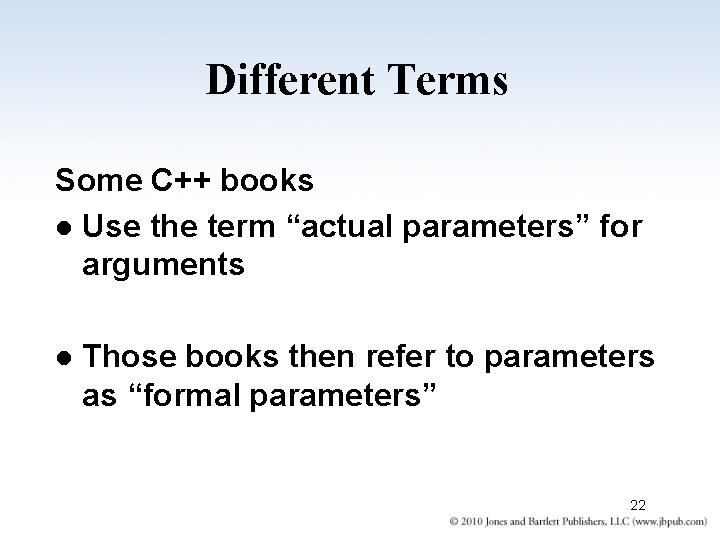Different Terms Some C++ books l Use the term “actual parameters” for arguments l Those books then refer to parameters as “formal parameters” 224000 25 age Argument in Calling Block Value Parameter Reference Parameter The value of the argument (25) is passed to the function when it is called The memory address (4000) of the argument is passed to the function when it is called In this case, the argument can be a variable identifier, constant, or expression In this case, the argument must be a variable identifier 23 23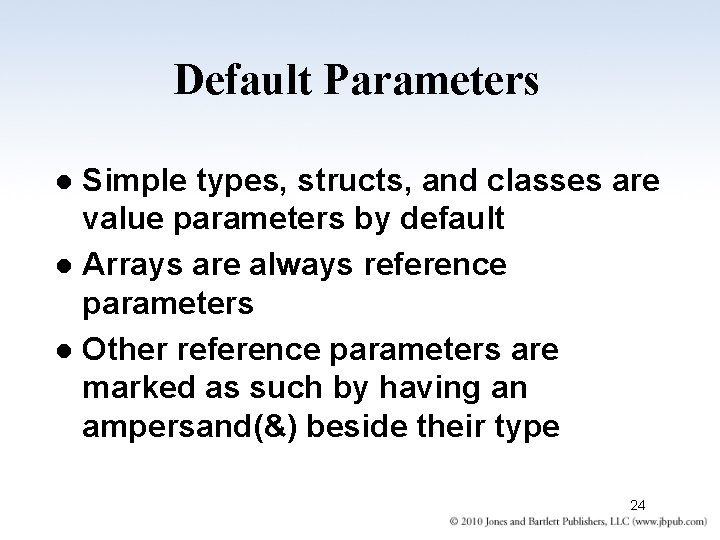Default Parameters Simple types, structs, and classes are value parameters by default l Arrays are always reference parameters l Other reference parameters are marked as such by having an ampersand(&) beside their type l 24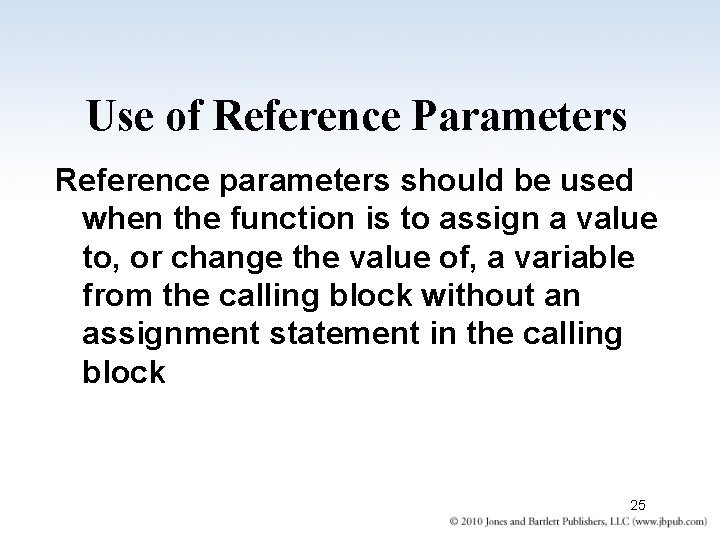Use of Reference Parameters Reference parameters should be used when the function is to assign a value to, or change the value of, a variable from the calling block without an assignment statement in the calling block 25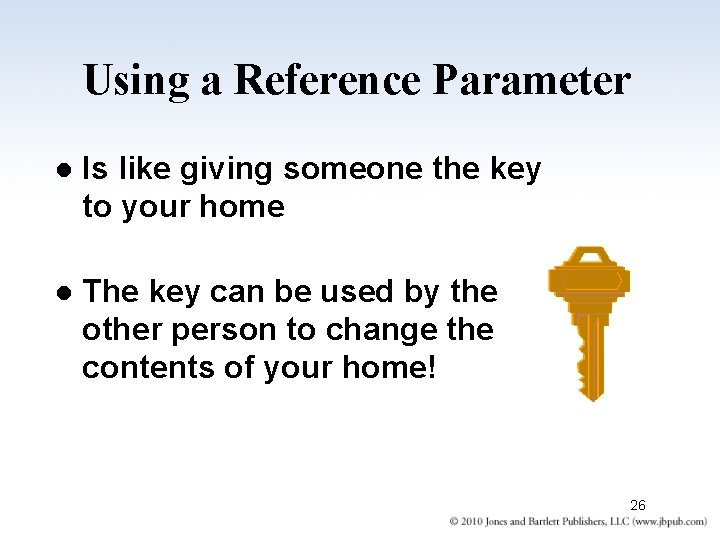Using a Reference Parameter l Is like giving someone the key to your home l The key can be used by the other person to change the contents of your home! 26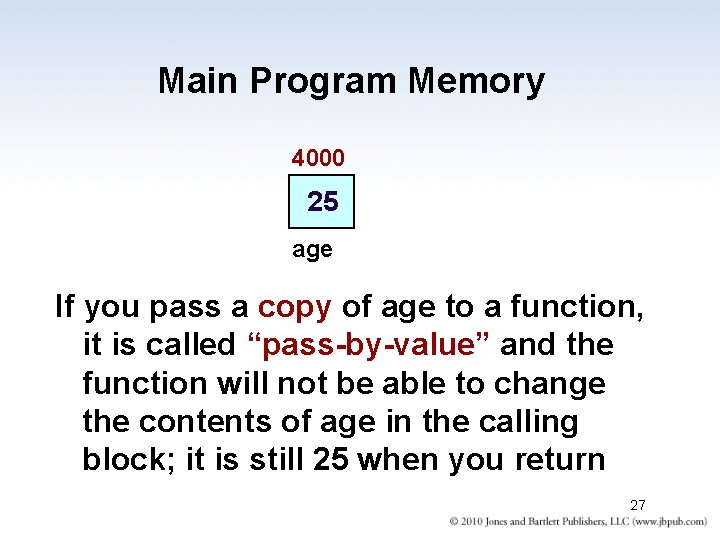Main Program Memory 4000 25 age If you pass a copy of age to a function, it is called “pass-by-value” and the function will not be able to change the contents of age in the calling block; it is still 25 when you return 27Main Program Memory 4000 25 age BUT, if you pass 4000, the address of age to a function, it is called “pass-by -reference” and the function will be able to change the contents of age in the calling block; it could be 23 or 90 when you return 28Additional Terms l Pass-by-reference is also called. . . n pass-by-address, or n pass-by-location Can you explain why? 29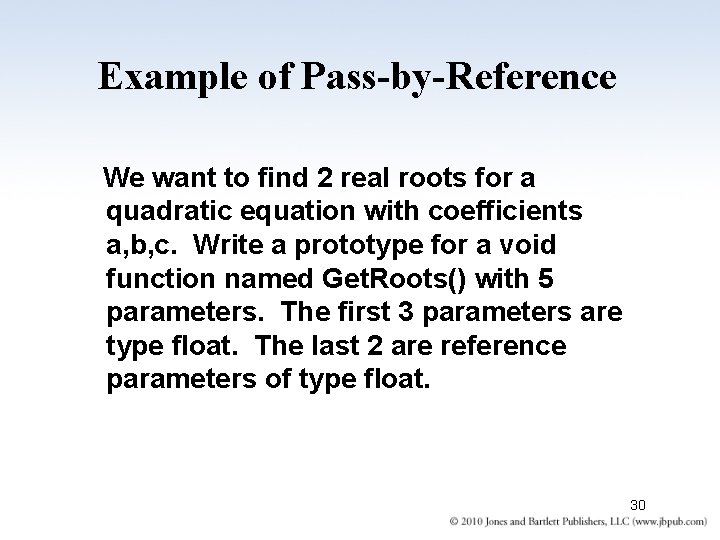Example of Pass-by-Reference We want to find 2 real roots for a quadratic equation with coefficients a, b, c. Write a prototype for a void function named Get. Roots() with 5 parameters. The first 3 parameters are type float. The last 2 are reference parameters of type float. 30// Prototype void Get. Roots(float, float&); Now write the function definition using this information This function uses 3 incoming values a, b, c from the calling block. It calculates 2 outgoing values root 1 and root 2 for the calling block. They are the 2 real roots of the quadratic equation with coefficients a, b, c. 31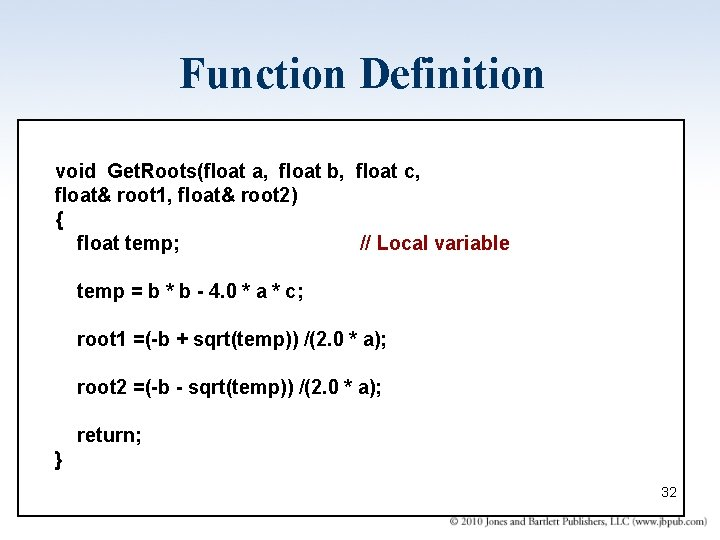Function Definition void Get. Roots(float a, float b, float c, float& root 1, float& root 2) { float temp; // Local variable temp = b * b - 4. 0 * a * c; root 1 =(-b + sqrt(temp)) /(2. 0 * a); root 2 =(-b - sqrt(temp)) /(2. 0 * a); return; } 32 32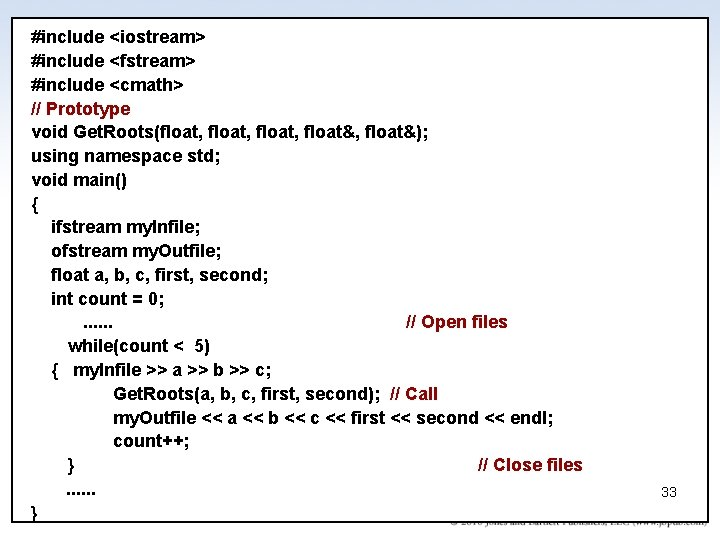#include <iostream> #include <fstream> #include <cmath> // Prototype void Get. Roots(float, float&); using namespace std; void main() { ifstream my. Infile; ofstream my. Outfile; float a, b, c, first, second; int count = 0; . . . // Open files while(count < 5) { my. Infile >> a >> b >> c; Get. Roots(a, b, c, first, second); // Call my. Outfile << a << b << c << first << second << endl; count++; } // Close files. . . } 33 33Pass-by-value “incoming” value of argument CALLING BLOCK FUNCTION CALLED 34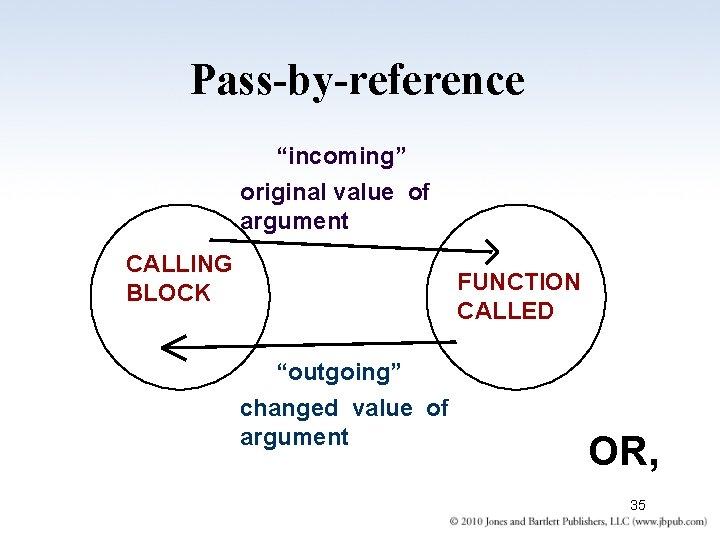Pass-by-reference “incoming” original value of argument CALLING BLOCK FUNCTION CALLED “outgoing” changed value of argument OR, 35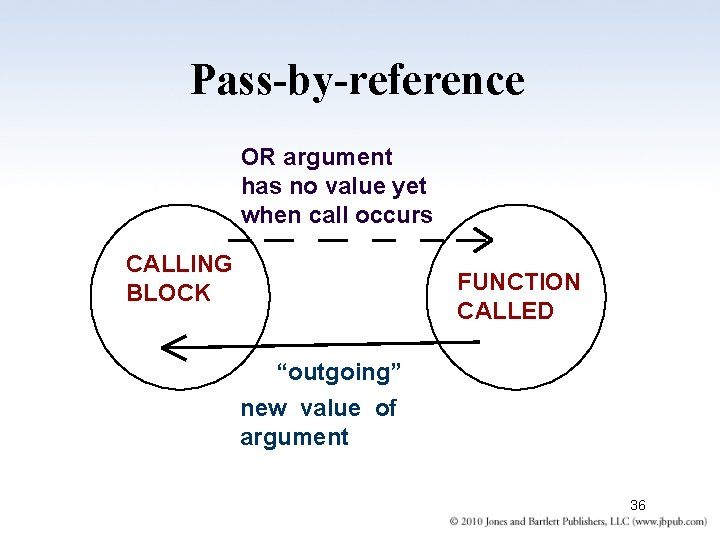Pass-by-reference OR argument has no value yet when call occurs CALLING BLOCK FUNCTION CALLED “outgoing” new value of argument 36Data Flow Determines Passing-Mechanism Parameter Data Flow Passing-Mechanism Incoming /* in */ Pass-by-value Outgoing /* out */ Pass-by-reference Incoming/outgoing Pass-by-reference /* inout */ 37Questions Why is a function used for a task? To cut down on the amount of detail in your main program (encapsulation) l Can one function call another function? Yes l Can a function even call itself? Yes, that is called recursion; it is very useful and requires special care in writing l 38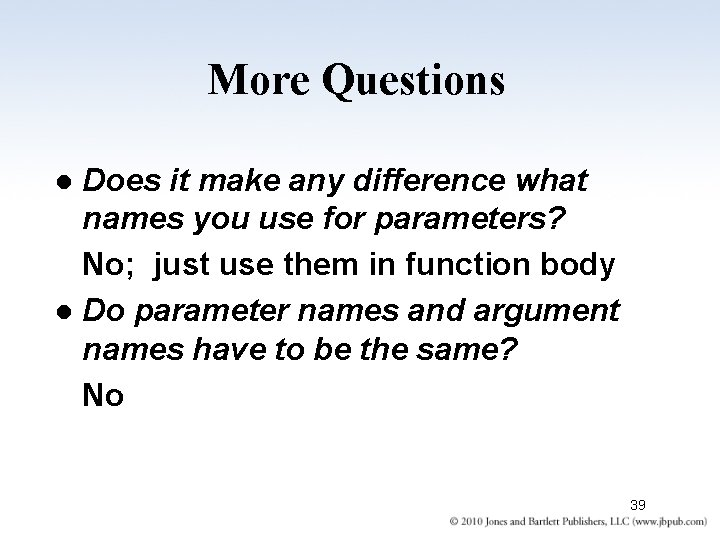More Questions Does it make any difference what names you use for parameters? No; just use them in function body l Do parameter names and argument names have to be the same? No l 39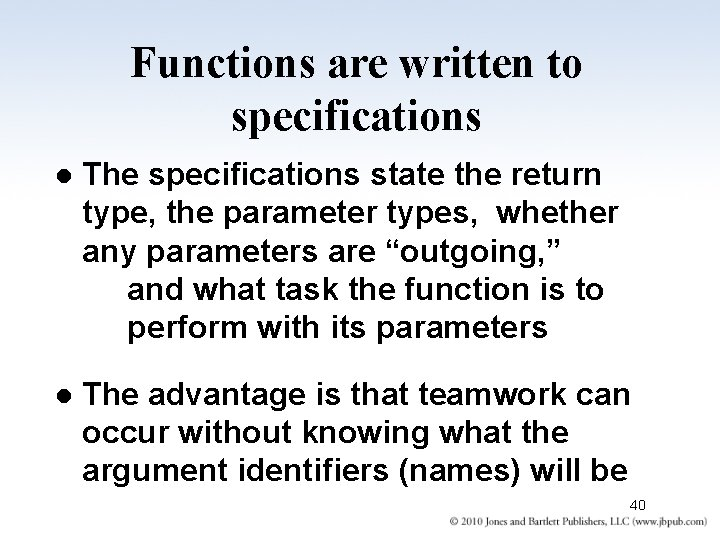Functions are written to specifications l The specifications state the return type, the parameter types, whether any parameters are “outgoing, ” and what task the function is to perform with its parameters l The advantage is that teamwork can occur without knowing what the argument identifiers (names) will be 40Write prototype and function definition for l A void function called Get. Rating() with one reference parameter of type char l The function repeatedly prompts the user to enter a character at the keyboard until one of these has been entered: E, G, A, P to represent Excellent, Good, Average, Poor 41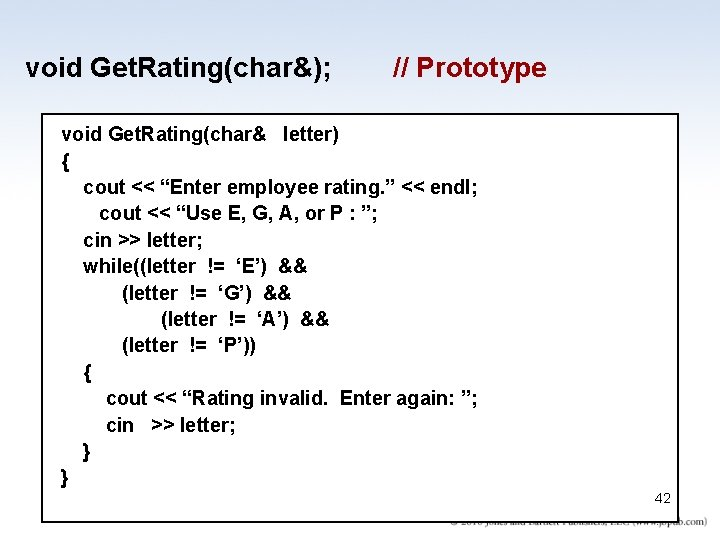void Get. Rating(char&); // Prototype void Get. Rating(char& letter) { cout << “Enter employee rating. ” << endl; cout << “Use E, G, A, or P : ”; cin >> letter; while((letter != ‘E’) && (letter != ‘G’) && (letter != ‘A’) && (letter != ‘P’)) { cout << “Rating invalid. Enter again: ”; cin >> letter; } } 42 42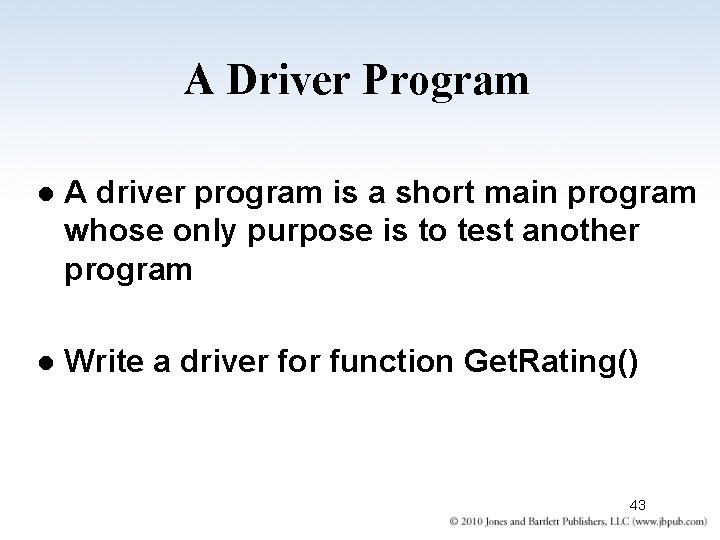A Driver Program l A driver program is a short main program whose only purpose is to test another program l Write a driver for function Get. Rating() 43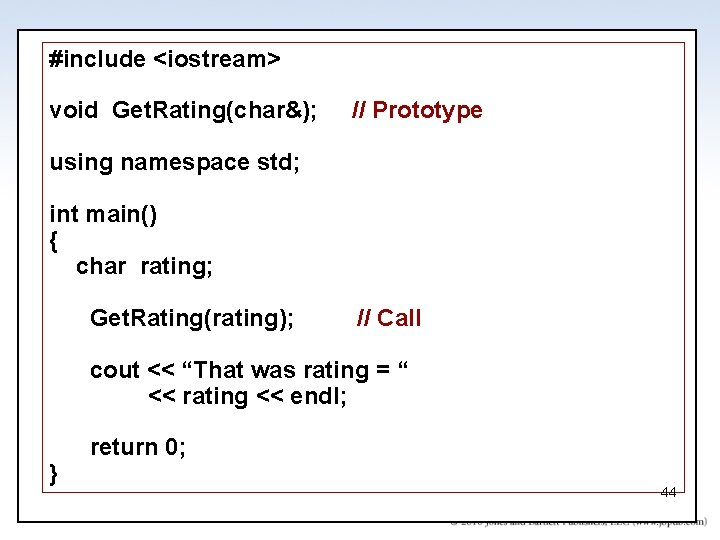#include <iostream> void Get. Rating(char&); // Prototype using namespace std; int main() { char rating; Get. Rating(rating); // Call cout << “That was rating = “ << rating << endl; } return 0; 44 44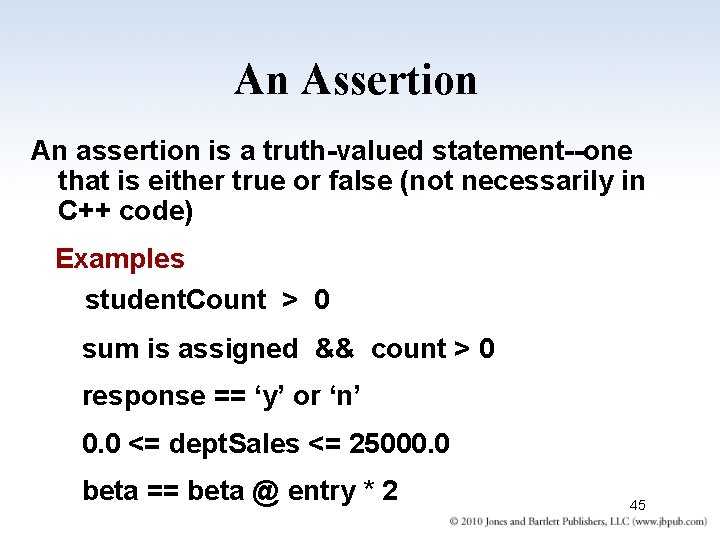An Assertion An assertion is a truth-valued statement--one that is either true or false (not necessarily in C++ code) Examples student. Count > 0 sum is assigned && count > 0 response == ‘y’ or ‘n’ 0. 0 <= dept. Sales <= 25000. 0 beta == beta @ entry * 2 45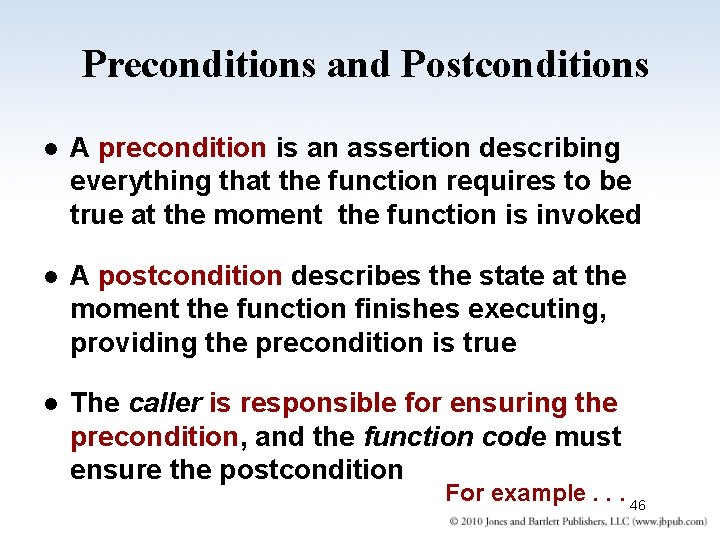Preconditions and Postconditions l A precondition is an assertion describing everything that the function requires to be true at the moment the function is invoked l A postcondition describes the state at the moment the function finishes executing, providing the precondition is true l The caller is responsible for ensuring the precondition, and the function code must ensure the postcondition For example. . . 46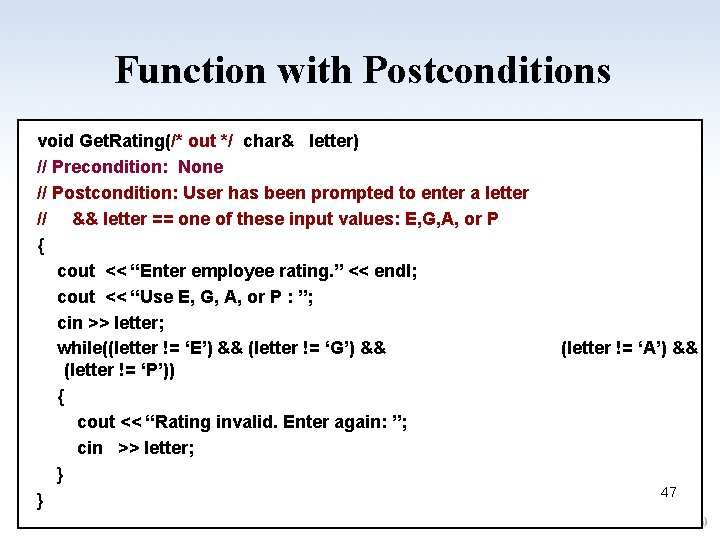Function with Postconditions void Get. Rating(/* out */ char& letter) // Precondition: None // Postcondition: User has been prompted to enter a letter // && letter == one of these input values: E, G, A, or P { cout << “Enter employee rating. ” << endl; cout << “Use E, G, A, or P : ”; cin >> letter; while((letter != ‘E’) && (letter != ‘G’) && (letter != ‘P’)) { cout << “Rating invalid. Enter again: ”; cin >> letter; } } (letter != ‘A’) && 47 47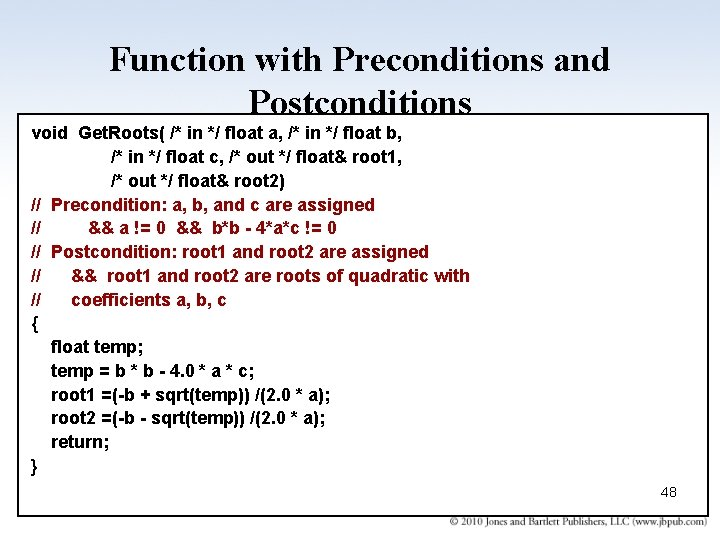Function with Preconditions and Postconditions void Get. Roots( /* in */ float a, /* in */ float b, /* in */ float c, /* out */ float& root 1, /* out */ float& root 2) // Precondition: a, b, and c are assigned // && a != 0 && b*b - 4*a*c != 0 // Postcondition: root 1 and root 2 are assigned // && root 1 and root 2 are roots of quadratic with // coefficients a, b, c { float temp; temp = b * b - 4. 0 * a * c; root 1 =(-b + sqrt(temp)) /(2. 0 * a); root 2 =(-b - sqrt(temp)) /(2. 0 * a); return; } 48 48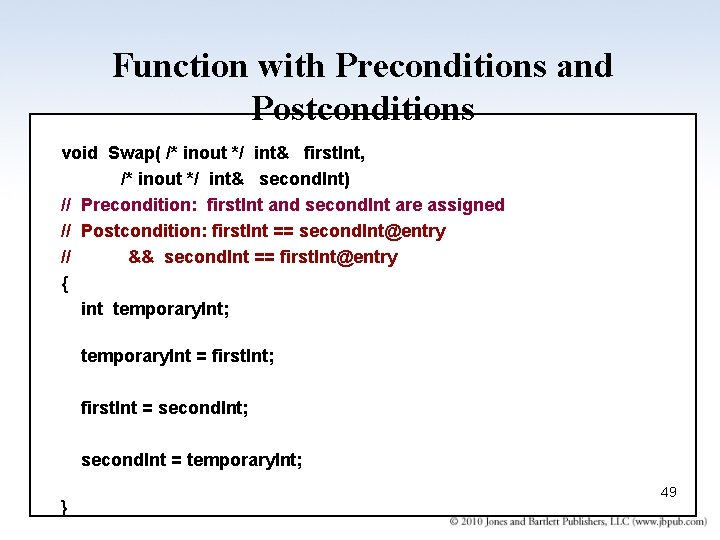Function with Preconditions and Postconditions void Swap( /* inout */ int& first. Int, /* inout */ int& second. Int) // Precondition: first. Int and second. Int are assigned // Postcondition: first. Int == second. [email protected] // && second. Int == first. [email protected] { int temporary. Int; temporary. Int = first. Int; first. Int = second. Int; second. Int = temporary. Int; } 49 49